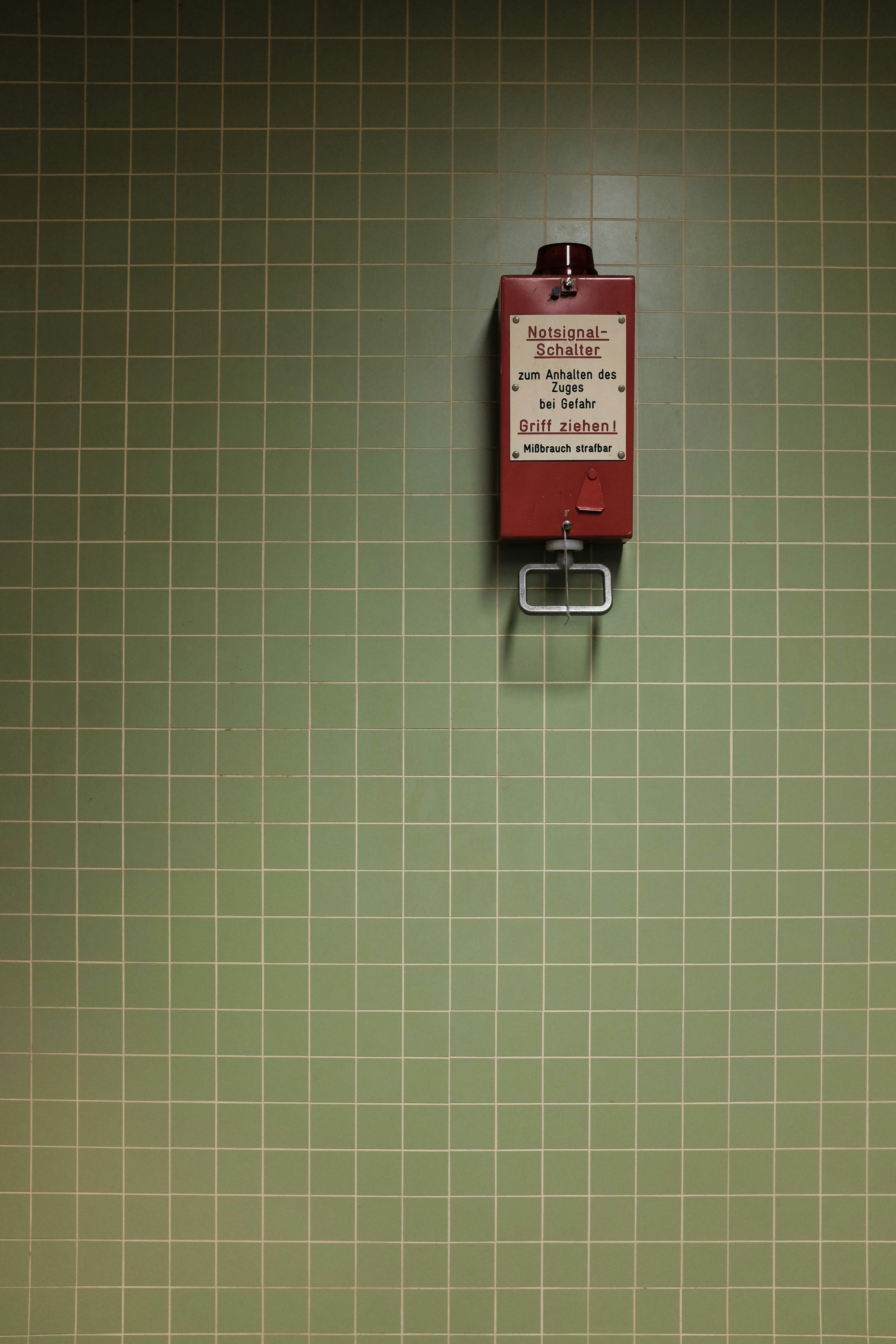Hackerrank - Grid Challenge Solution

# Hackerrank - Grid Challenge SolutionGiven a square grid of characters in the range ascii[a-z], rearrange elements of each row alphabetically, ascending. Determine if the columns are also in ascending alphabetical order, top to bottom. Return YES if they are or NO if they are not.

For example, given:

a b c
a d e
e f g


The rows are already in alphabetical order. The columns a a e, b d f and c e g are also in alphabetical order, so the answer would be YES. Only elements within the same row can be rearranged. They cannot be moved to a different row.

Function Description

Complete the gridChallenge function in the editor below. It should return a string, either YES or NO.

gridChallenge has the following parameter(s):

• grid: an array of strings

Input Format

The first line contains , the number of testcases.

Each of the next  sets of lines are described as follows:
- The first line contains , the number of rows and columns in the grid.
- The next  lines contains a string of length

Constraints

Each string consists of lowercase letters in the range ascii[a-z]

Output Format

For each test case, on a separate line print YES if it is possible to rearrange the grid alphabetically ascending in both its rows and columns, or NO otherwise.

Sample Input

1
5
ebacd
fghij
olmkn
trpqs
xywuv


Sample Output

YES


Explanation

The x grid in the  test case can be reordered to

abcde
fghij
klmno
pqrst
uvwxy


This fulfills the condition since the rows 1, 2, ..., 5 and the columns 1, 2, ..., 5 are all lexicographically sorted.

### Solution in Python

def gridChallenge(arr):
for j in range(len(arr)):
for i in range(1,len(arr)):
if arr[i][j]<arr[i-1][j]:
return "NO"
return "YES"
for _ in range(int(input())):
arr = [sorted(input()) for i in range(int(input()))]
print(gridChallenge(arr))